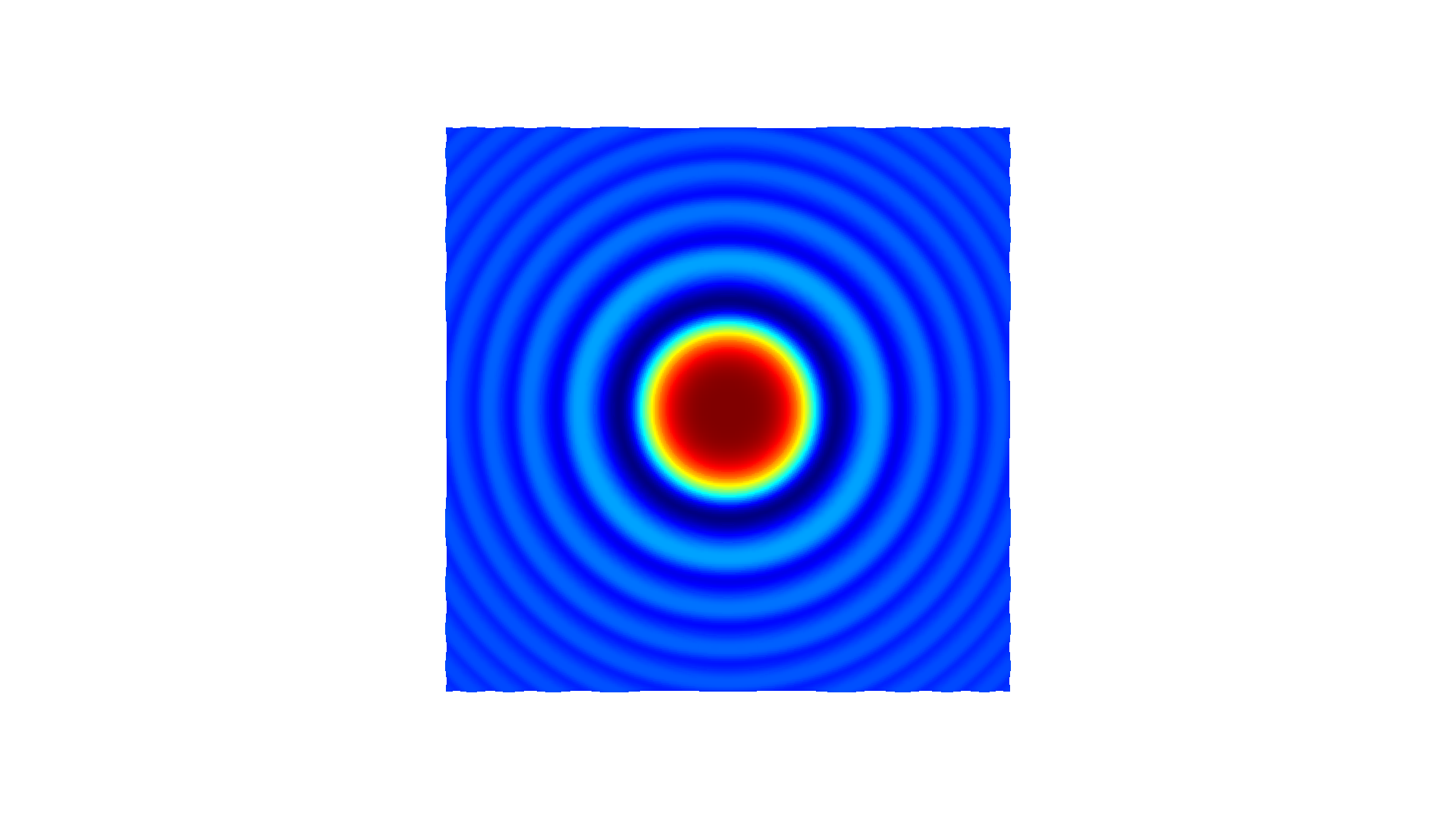# Working with NumPy¶

All data structures in Open3D are natively compatible with a NumPy buffer. The following tutorial generates a variant of sync function using NumPy and visualizes the function using Open3D.

First, we generate a $$n \times 3$$ matrix xyz. Each column has $$x$$, $$y$$, and $$z$$ values of the function $$z = \frac{sin(x^2 + y^2)}{x^2 + y^2}$$. $$z_\text{norm}$$ is the normalized map of $$z$$ for the [0,1] range.

:

# Generate some neat n times 3 matrix using a variant of sync function
x = np.linspace(-3, 3, 401)
mesh_x, mesh_y = np.meshgrid(x, x)
z = np.sinc((np.power(mesh_x, 2) + np.power(mesh_y, 2)))
z_norm = (z - z.min()) / (z.max() - z.min())
xyz = np.zeros((np.size(mesh_x), 3))
xyz[:, 0] = np.reshape(mesh_x, -1)
xyz[:, 1] = np.reshape(mesh_y, -1)
xyz[:, 2] = np.reshape(z_norm, -1)
print('xyz')
print(xyz)

xyz
[[-3.         -3.          0.17846472]
[-2.985      -3.          0.17440115]
[-2.97       -3.          0.17063709]
...
[ 2.97        3.          0.17063709]
[ 2.985       3.          0.17440115]
[ 3.          3.          0.17846472]]


## From NumPy to open3d.PointCloud¶

Open3D provides conversion from a NumPy matrix to a vector of 3D vectors. By using Vector3dVector, a NumPy matrix can be directly assigned to open3d.PointCloud.points.

In this manner, any similar data structure such as open3d.PointCloud.colors or open3d.PointCloud.normals can be assigned or modified using NumPy. The code below also saves the point cloud as a ply file for the next step.

:

# Pass xyz to Open3D.o3d.geometry.PointCloud and visualize
pcd = o3d.geometry.PointCloud()
pcd.points = o3d.utility.Vector3dVector(xyz)
o3d.io.write_point_cloud("sync.ply", pcd)

:

True


## From open3d.PointCloud to NumPy¶

As shown in this example, pcd_load.points of type Vector3dVector is converted into a NumPy array using np.asarray.

:

# Load saved point cloud and visualize it

# Convert Open3D.o3d.geometry.PointCloud to numpy array

xyz_load